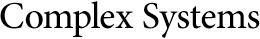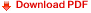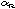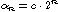## Scaling of Preimages In Cellular AutomataErica Jen
Theoretical Division, MS-B258, Los Alamos National Laboratory,
Los Alamos, NM 87545, USA

#### Abstract

A cellular automaton consists of a lattice of sites whose values evolve deterministically according to a local interaction rule. For a given rule and arbitrary spatial sequence, the preimage of the sequence is defined to be the set of tuples that are mapped by the rule onto the sequence. Recurrence relations are provided that express the number of preimages for a general spatial sequence in terms of the number of preimages for its subsequences. These relations are applied to the analysis of a quantitydefined as the total number of preimages for spatial sequences of lengthwhose probabilities of occurrence are in a certain sense minimal after one iteration of the rule. In particular, the recurrence relations are used to characterize automata rules by parameters representing the amount of information about an arbitrary spatial sequence needed to determine the values that, when appended to the sequence, minimize the number of preimages. On the basis of this characterization, it is proved that, for all nearest-neighbor automata rules on infinite lattices, the quantityscales exactly with the lengthof the spatial sequence; that is,forsufficiently large, whereis a constant depending on the rule. A symmetric result holds in the case of maximal preimages.